I.3.3

4/18/2021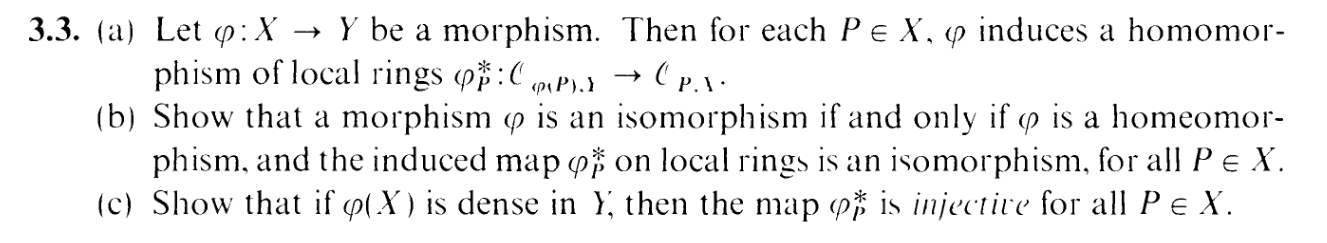If you are having trouble reading that, it says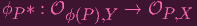"Yo, this notation is getting kinda complicated." I know, right. I'm barely hanging on as well. Here's what all that nonsense means: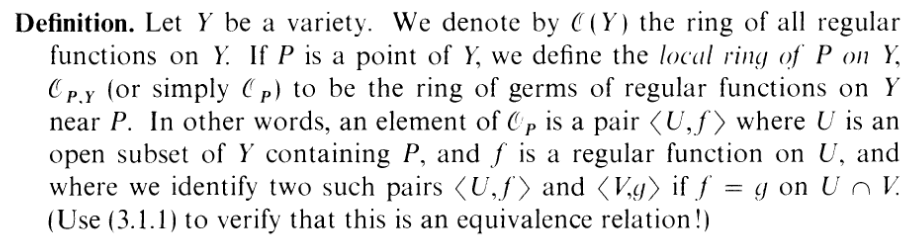"Ah, the ring of of germs of regular functions. I see... It all makes sense now..." That's the spirit, reader. Fake it till you make it. And don't worry, I did not use (3.1.1) to verify that this is an equivalence relation! You what they say: "To use it, you have to make an ass out of u and me"... What?

PART (a):

The idea for this whole exercise is not to be insightful and thoughtful about the meaning of what's happening, but to just plunge into the symbols and meme your way through it. In mathematics, we call these exercises "computational." I prefer to call them symbo bimbo bashes (whose adjectivalization is "symbo bimbo bashful". Make sure to memorize this: I will test you on this in a future post), as I, by virtue of experiencing qualia and all that, am above the mere manipulation of formal symbols and can find certain combinations of words more amusing and vivid than others, although I have been referred to in the past as "not human". Now, remember, we already know that ϕ : X Y induces a morphism that looks like this:

 ϕ* :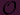(Y ) →(X) (f : U → k)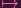(f ∘ ϕ : ϕ-1(U) → k)

which coaxes us into defining ϕP* as

 ϕP* :ϕ(P),Y →P,X < U,f > →< ϕ-1(U),ϕ * (f) > =< ϕ-1(U),f ∘ ϕ >

Our job is show that this is well defined. So suppose < U,f >=< V,g > inϕ(P),Y . Then

 ϕP * (< U,f >) =< ϕ-1(U),f ∘ ϕ > =< ϕ-1(U) ∩ ϕ-1(V ),f ∘ ϕ > =< ϕ-1(U ∩ V ),f ∘ ϕ > =< ϕ-1(U ∩ V ),g ∘ ϕ > (since f = g on U ∩ V ) =< ϕ-1(U) ∩ ϕ-1(V ),g ∘ ϕ > =< ϕ-1(V ),g ∘ ϕ > = ϕP * (< V,g >)

so yea, it's well-defined. And the ring homomorphism properties are trivial. Done.

PART (b):Starting with the "only if". Suppose ϕ an isomorphism. Then ϕ is automatically a homeomorphism. And ϕP-1* is clearly and inverse for ϕP*. So that direction is easy...

For the other direction, note that all we need to do is show that ϕ-1 satisfies the regularity property. I.e. given U, open in X and f : U k a regular function, is f ϕ-1 : ϕ(U) k regular?

Time for some symbo bimbo bashing. Since ϕP* is an isomorphism, we have access to the inverse map ϕP-1* :P,Xϕ(P),Y for any P X. Let P U. Then since < U,f >P,X, we have that ϕP-1 * (< U,f >) =< ϕ(U),f ϕ-1 > is inϕ(P),Y . I.e. f ϕ-1 is regular. DONE.

PART (c):A bit tougher than the first two parts. So let's say ϕP* (< U,f >) = ϕP* (< V,g >). I want to show that < U,f >=< V,g >, i.e. that f = g on U V .

First thing, let's unwrap the given equality:

 ϕP * (< U,f >) = ϕP * (< V,g >)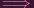< ϕ-1(U),f ∘ ϕ > =< ϕ-1(V ),g ∘ ϕ >f ∘ ϕ > = g ∘ ϕ > (on ϕ-1(U ∩ V ))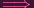f = g (on U ∩ V ∩ ϕ(X))

The key point is that we are basically given that f = g on a significant subset S = U V ϕ(X) of U V . In fact, since ϕ(X) is dense in Y , this subset should be dense on U V shouldn't it? It's been a while since we've invoked our fave closure property on this blog. Let's use it!

 clU∩V (S) = U ∩ V ∩ clY ϕ(X) = U ∩ V ∩ Y = U ∩ V

Yep!

So f = g on "almost all" of U V . What about the points that are not in S? Like, let's say R was a point in U V but not in S. We want f = g on R as well.

Well, since f and g are regular let's say that W is a neighborhood of R such that f = f1∕f2 and g = g1∕g2 are rational. Let's set h to be the cross-multiplication of those polynomials,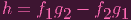so, h ϕ = 0 at least on S, a dense subset of U V . Around this point, I was reminded of something that popped up earlier in the text: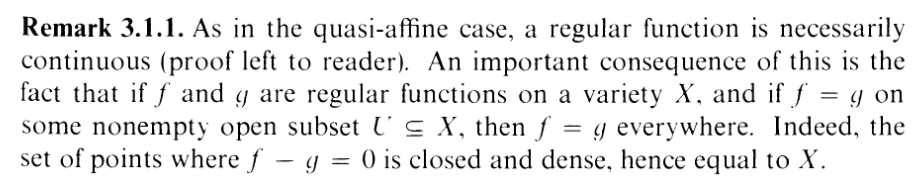Ooohh. It's.. (3.1.1), haha. And I will use it here. Not directly, but using the logic as inspiration. You see, asking "where is h = 0 in U V is the same as computing Z(h) (U V ). Now, from our work we know that

 Z(h) ∩ (U ∩ V ) ⊃ S

So what happens if we take the closure in U V on both sides? We get

 Z(h) ∩ (U ∩ V ) ⊃ U ∩ VZ(h) ∩ (U ∩ V ) = U ∩ V

(why did the left side stay the same? Cause by the definition of the subspace topology, it's a closed subset of U V , so taking the closure does nothing). Hence h = 0 on all of U V , including that pesky point R. So f(R) = g(R), as needed. FIN.

And just in case you're wondering, I am too lazy to figure out how to make the sheaf symbolsturn out pink. You know what? They might as well stay black for the entire rest of the blog. We'll call it an inside joke, just like the long gaps and delays between exercises are an "inside joke".